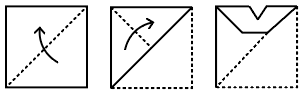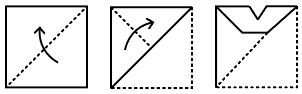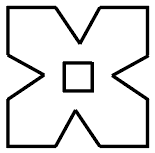Question 3

# The sequence of folding a piece of paper and the manner in which the folded paper has been cut is shown in the following figures. How would this paper look when unfolded?Solution

Given that The sequence of folding a piece of paper and the manner paper cut given belowfrom the above figure if we can unfold the figure we can obtain the given belowtherefore Option (C) Ans